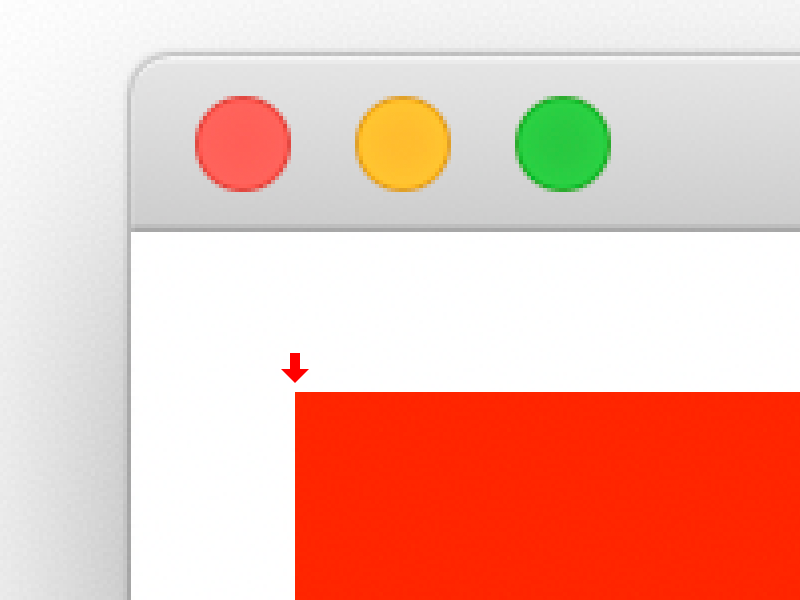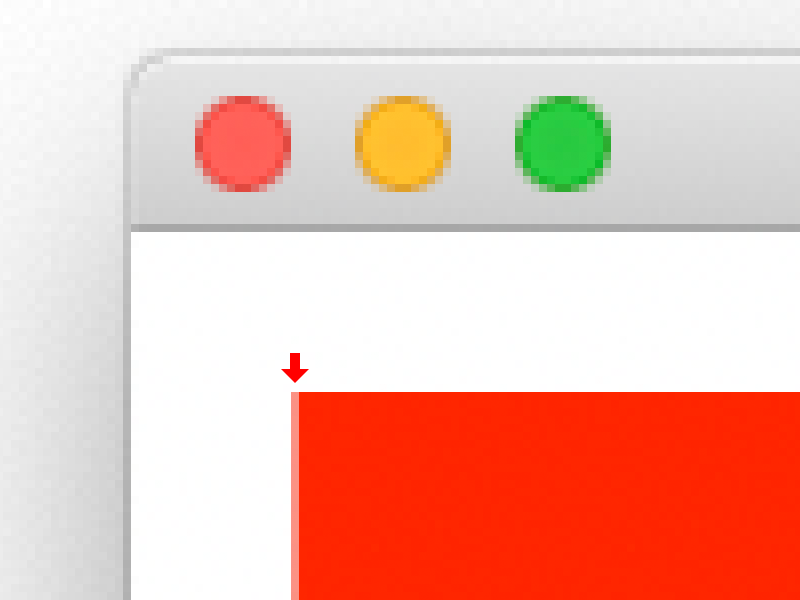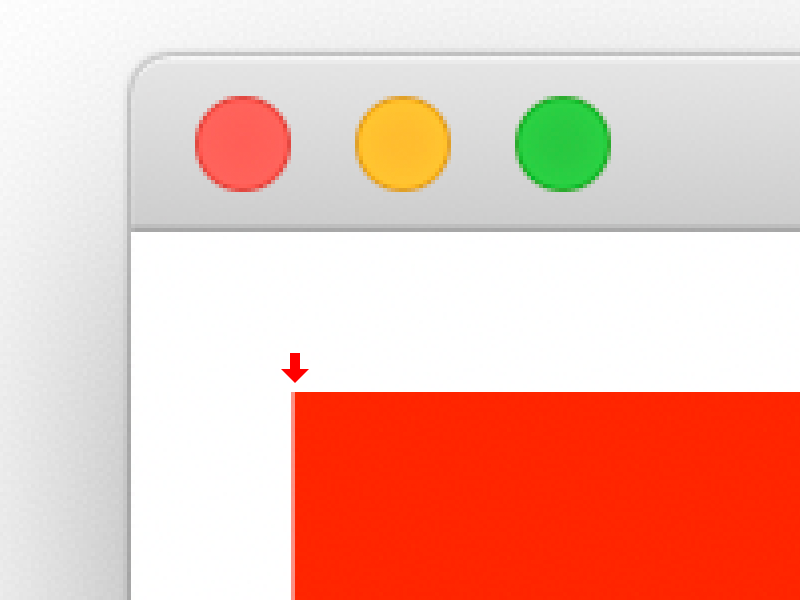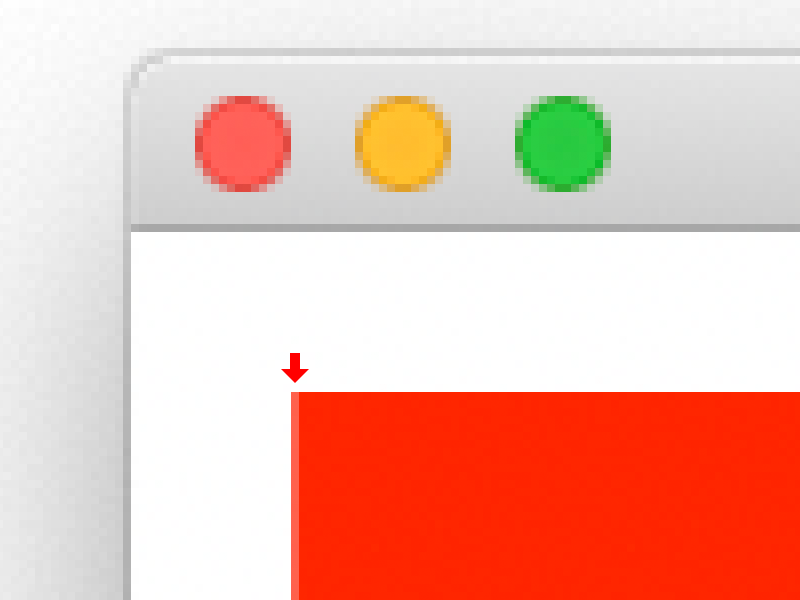In my previous post I mentioned (in code) rounding drawing coordinates by a factor evenly divisible by the graphics object's scaling factor. In this post, my goal is explain what problem this is trying to solve, and of course solve it.

You might think "oh I can just use doubles now and not worry about rounding" and while you're correct, you'll introduce a level of blurriness that's not immediately obvious. Your graphics will look ok, but will probably look worse than when you were using integers. Here's some examples, blown up to show detail.

The first example is a rectangle drawn at X=20.5 on a 2x screen. It is nice a perfectly sharp edge because 20.5 x 2 is 41, so it is drawn precisely 41 pixels from the left.The second example is the exact same code, except rendered on a 1x screen. The left edge is now blended with the white pixels before it. This is because 20.5 x 1 is still 20.5, but there's no such thing as half a pixel. So the graphics object has to blend the two colors together to approximate what it was asked to do. In this case, 50% of the left color (white) and 50% of the right color (red.)If you think this is just a problem for 1x screens though, think again. In this next example, we're going to draw the same shape at 20.25 instead. First up is the 2x screen:Just like before, because the point-to-pixel math does not result in a whole number, Xojo has to estimate again.

And on a 1x screen:Wait... that doesn't look half bad. The effect is still there, but it's not very noticeable. This is because at 20.25, Xojo needs only 25% white and 75% red. So it still looks pretty red.

The point is though, if you want sharp results, you'll need coordinates that match the screen you're drawing too.

### The solution

What we need is a function to round to the nearest whole on 1x screens, nearest half on 2x screens, and third on 3x screens. Plus of course any other density screen that may come along, as well as the crazy in-between choices that Windows gives users.

Performing the rounding is simple, just round the value divided by the factor, then multiply that result by the factor.

The whole function could look like:

``````Public Function NearestMultiple(Value As Double, Factor As Double) As Double
// If this is already a whole number, there's no reason for more math.
Var Whole As Integer = Floor(Value)
If Whole = Value Then
Return Value
End If

Return Round(Value * Factor) / Factor
End Function``````

With this in hand, we can now scale this X=20.5 value to get pixel-perfect drawing no matter what:

``````// 21 on 1x, 20.5 on 2x, 20.6666 on 3x.
Var Left As Double = NearestMultiple(20.5, G.ScaleX)``````

And for our 20.25 value:

``````// 20 on 1x, 20.5 on 2x, 20.3333 on 3x.
Var Left As Double = NearestMultiple(20.25, G.ScaleX)``````